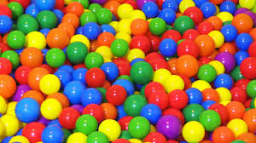# Probability 68584

There are five whites and nine blacks in the destiny. We will choose three balls at random. What is the probability that
a) the selected balls will not be the same color,
b) will there be at least two blacks between them?

p1 =  0.7418
p2 =  0.7253

### Step-by-step explanation:Did you find an error or inaccuracy? Feel free to write us. Thank you!

Tips for related online calculators
Need help calculating sum, simplifying, or multiplying fractions? Try our fraction calculator.
Would you like to compute the count of combinations?